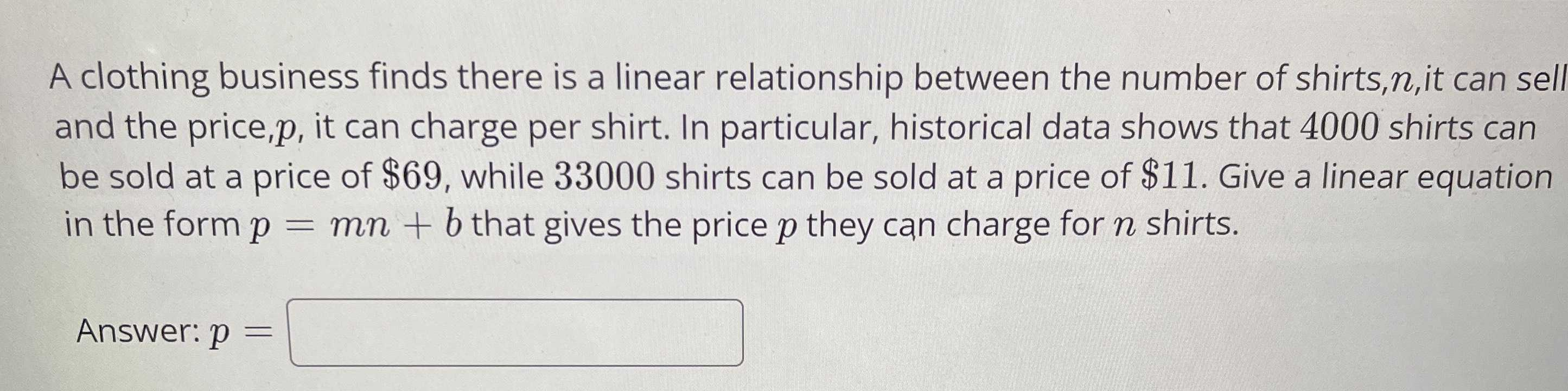### ¿Todavía tienes preguntas de matemáticas?

Pregunte a nuestros tutores expertos
Algebra
PreguntaA clothing business finds there is a linear relationship between the number of shirts, $$n$$ , it can sell and the price, $$p$$ , it can charge per shirt. In particular, historical data shows that $$4000$$ shirts can be sold at a price of $$\ 69$$ , while $$33000$$ shirts can be sold at a price of $$\ 11$$ . Give a linear equation in the form $$p = m n + b$$ that gives the price $$p$$ they can charge for $$n$$ shirts. Answer: $$p = \square$$